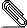# Re: Formatting numbered equations

```Am 19.04.2010 20:25, schrieb Marshall Feldman:

```
```I have several questions regarding numbered equations:
```
```
```
At first, please have a look at LyX's Math manual that you find in LyX's help menu. This will give you many info and answers.
```
```
```1. How does one add punctuation to numbered equations?
```
```
What do you mean here?

```
```2. How does one make the equations be part of a paragraph that begins
before and continues after them?
```
```
```
I also don't understand what you mean here. If you are in a paragraph and press Ctrl+Shift+m you get a displayed formula that is part of this paragraph. (If you want to have an inline equation, press only Ctrl+m (omit the Shift key).)
```
```
```3. How does one continue a numbered equation across multiple lines?
```
```>
```
```2 + 2 = 4 (1),
C = 2 x pi x r (2), and
A = (1/2) b x h (3).

In the above example, I can't figure out how to add the commas, period,
and conjunction adjacent to the equation numbers.
```
```
```
The equation number is the last character of an equation line, according to ISO norms. So you need to place the phrase "add" into a separate line. This can be done by either
```
- using the command \intertext, as described in the Math manual
- use two equations as in the attached example file

The latter is the usual method.

```
(By the way in English there is no comma before the "and" if the part after the "and" is the last enumeration in a sentence; like in
```"A, B, C and D are letters.")

```
```Also notice that the
sentence beginning with "As" is part of the paragraph beginning with
"Three."
```
```
```
Everything is one paragraph if you don't press Enter after of before the equations. In the attached file there is only one paragraph.
```
```
```Regarding my third question, I have a long equation that should span
multiple lines. Instead, LyX keeps it on one line that runs off the
right side of the page, when it should look like:

5 = 1 + 5 = 2 + 3 = 3 + 2 = 4 + 1 = 10/2
= 50/10 = 100/20 (4)
```
```
```
You are responsible for line breaks in equations. Possible methods are described in the Math manual.
```
regards Uwe
```newfile3.lyx
Description: application/lyx